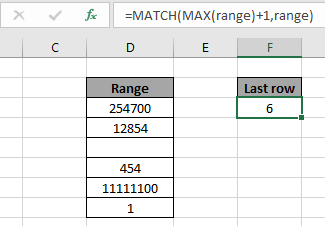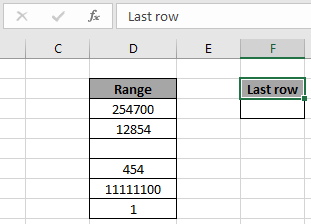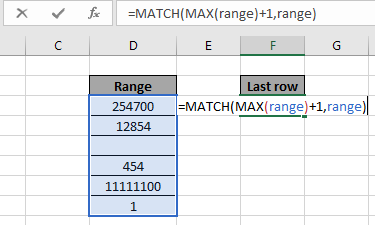# Find the last row of data with numbers in ExcelIn this article, we will learn to Find the Last ROW of numbers data in Excel.

Scenario:

In simple words, while working with long range of numbers data, we need to extract the last non blank cell using the formula in Excel.

How to solve the problem?

For this article we will be needing the use the following functions:

Use the formula:

= MATCHMAX ( range ) + 1 , range )

range: list of numbers only numbers values and blank cells.

Example:

All of these might be confusing to understand. So, let's test this formula via running it on the example shown below.

Here we have a list of numbers values and we need to find the last non blank cell row ignoring blank cells.Now we will use the below formula to get the last non blank cell
Formula:

= MATCHMAX ( range ) + 1 , range )

Range: Named range used for the range D3 : D8.

Explanation:

• MAX function finds the MAX value of the numbers and we added + 1 to it.
• Now the MATCH function finds the returned number in range.
• And MATCH function wouldn't be able to find the number as the lookup_value is greater than the MAX value in the array.
• So the MATCH function reaches the last value for the lookup_value and returns the last row number instead.Here the array to the function is given as named range and cell as cell_reference. Press Enter to get the result.As you can see in the above snapshot the row number of the last non blank cell is 6.

Here are all the observational notes regarding using the formula.

Notes:

1. The formula only works with numbers only.
2. Operators like equals to ( = ), less than equal to ( <= ), greater than ( > ) or not equals to ( <> ) can be performed within function applied with numbers only.

Hope this article about how to find the last row of data with numbers in Excel is explanatory. Find more articles on COUNTIF functions here. If you like our blogs, share it with your on Facebook. And also you can follow us on Twitter and Facebook. We would love to hear from you, do let us know how we can improve, complement or innovate our work and make it better for you. Write to us at info@exceltip.com

Related Articles

Find the last row of data with numbers in Excel : In a range of text values find the last of data in excel.

How to use the SUMPRODUCT function in Excel: Returns the SUM after multiplication of values in multiple arrays in excel.

COUNTIFS with Dynamic Criteria Range : Count cells dependent on other cell values in Excel.

COUNTIFS Two Criteria Match : Count cells matching two different criteria on list in excel.

COUNTIFS With OR For Multiple Criteria : Count cells that have multiple criteria match using the OR function.

COUNTIFS Function in Excel : Count cells dependent on other cell values.

How to Use Countif in VBA in Microsoft Excel : Count cells using Visual Basic for Applications code.

How to use wildcards in excel : Count cells matching phrases using the wildcards in excel

Popular Articles

50 Excel Shortcut to Increase Your Productivity : Get faster at your task. These 50 shortcuts will make you work even faster on Excel.

How to use the VLOOKUP Function in Excel : This is one of the most used and popular functions of excel that is used to lookup value from different ranges and sheets.

How to use the COUNTIF function in Excel : Count values with conditions using this amazing function. You don't need to filter your data to count specific values. Countif function is essential to prepare your dashboard.

How to Use SUMIF Function in Excel : This is another dashboard essential function. This helps you sum up values on specific conditions.

Terms and Conditions of use

The applications/code on this site are distributed as is and without warranties or liability. In no event shall the owner of the copyrights, or the authors of the applications/code be liable for any loss of profit, any problems or any damage resulting from the use or evaluation of the applications/code.